Work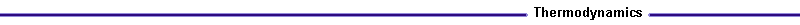Figure 3. Work done on the piston due to pressure P Introduction The work produced is due to the gas pressure on the piston. Figure 3 represents the process the piston experiences. Assuming that the pressure in the crank case is atmospheric, then the gas pressure will be relative to the crank case pressure. P = Pgas - Pcrank case For a small displacement, dx, the work is dW. The following relationship for dW can be developed: dW = F dx = PA dx = P dV For a finite volume change, work is given by: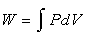The work is can also be represented per unit mass of fuel and air, the specific work.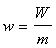The specific volume is also given by: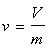This work, W, is called the indicated work and will be represented as Wi. Friction in the rings and bearings are included with a friction work term, Wf. The work at the crank shaft is the brake work, Wb. The brake work is defined as the indicated work less the friction work. Wb = Wi - Wf The mechanical efficiency,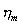, is defined as:= Wb/Wi = 1 - Wf/Wi Mean Effective Pressure The pressure in the cylinder changes during the expansion stroke. First increasing due to the heat addition, and then decreasing due to the increase in cylinder volume. We can define a mean effective pressure to determine the work.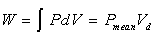A mean effective pressure can be found for the indicated, friction and brake work. Pmean,i = Wi/Vd (i = indicated) Pmean,f = Wf/Vd (f = friction) Pmean,b = Wb/Vd (b = brake) A naturally aspirated Otto cycle engine has a Pmean,b ~ 1000 kPa. If turbo charged, the engine Pmean,b can increase to above 1500 kPa.